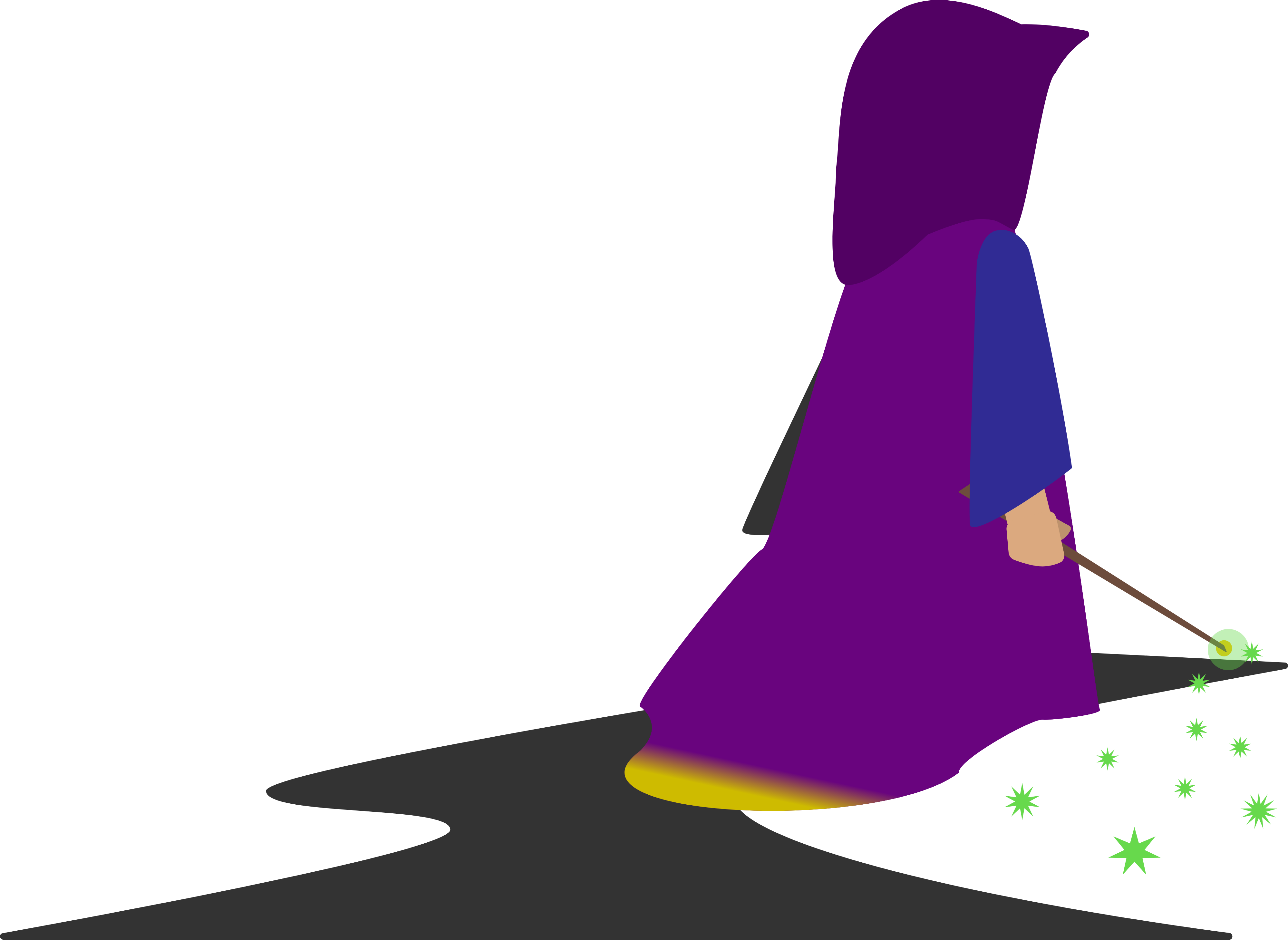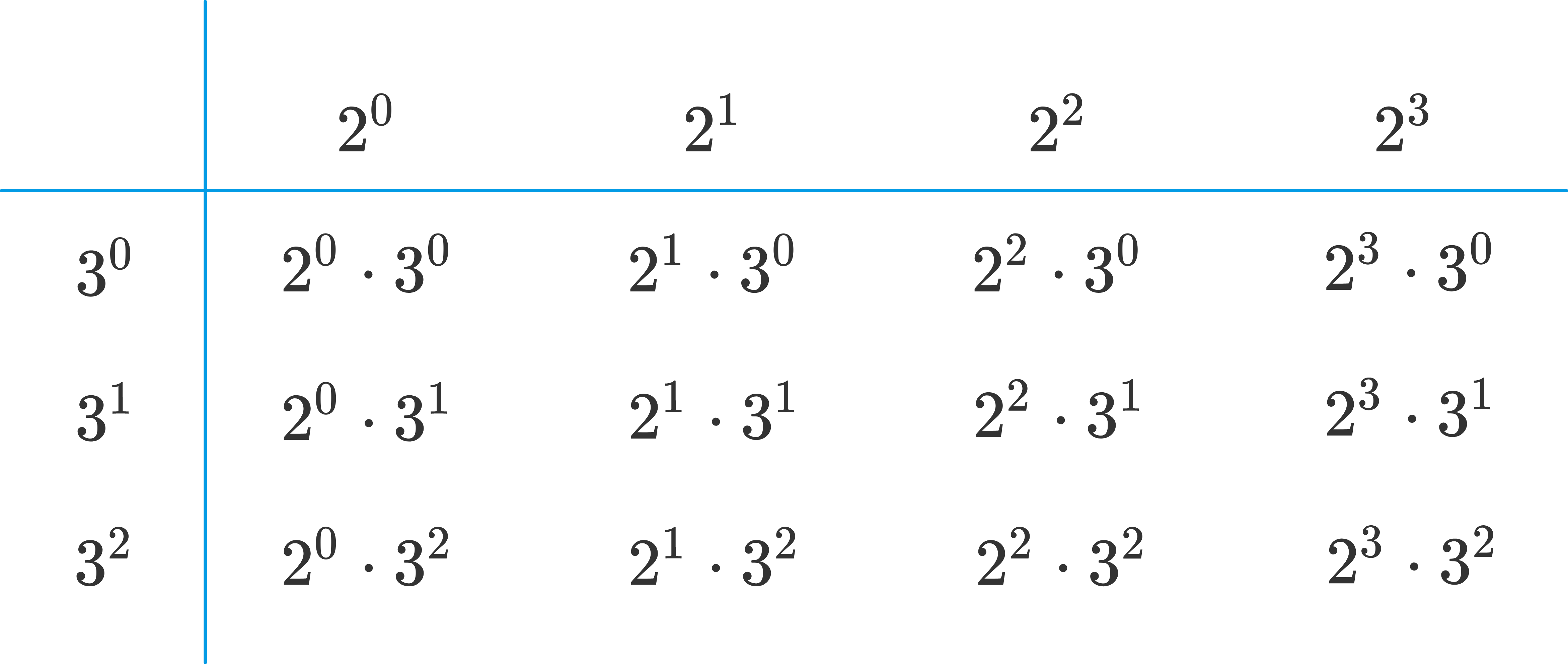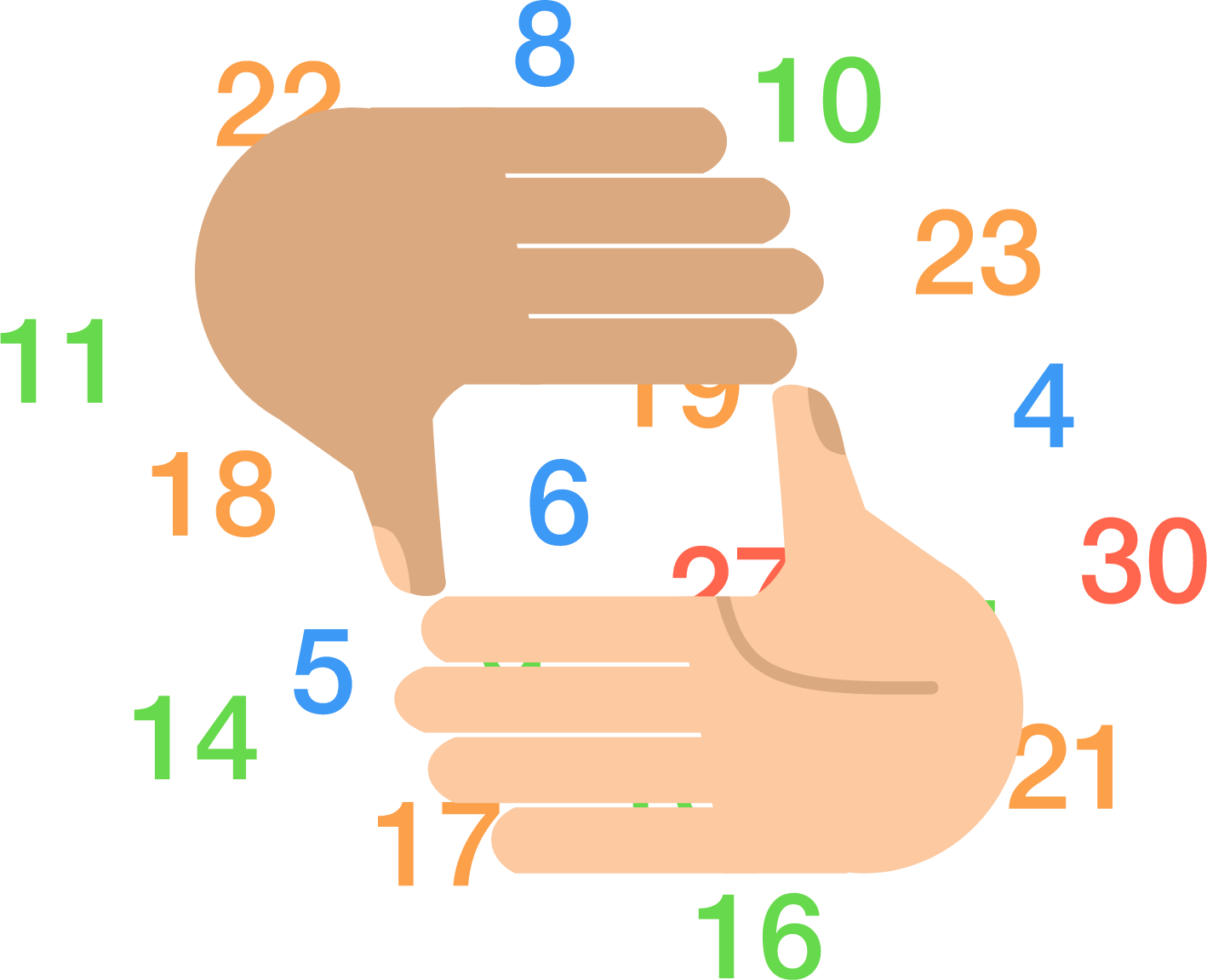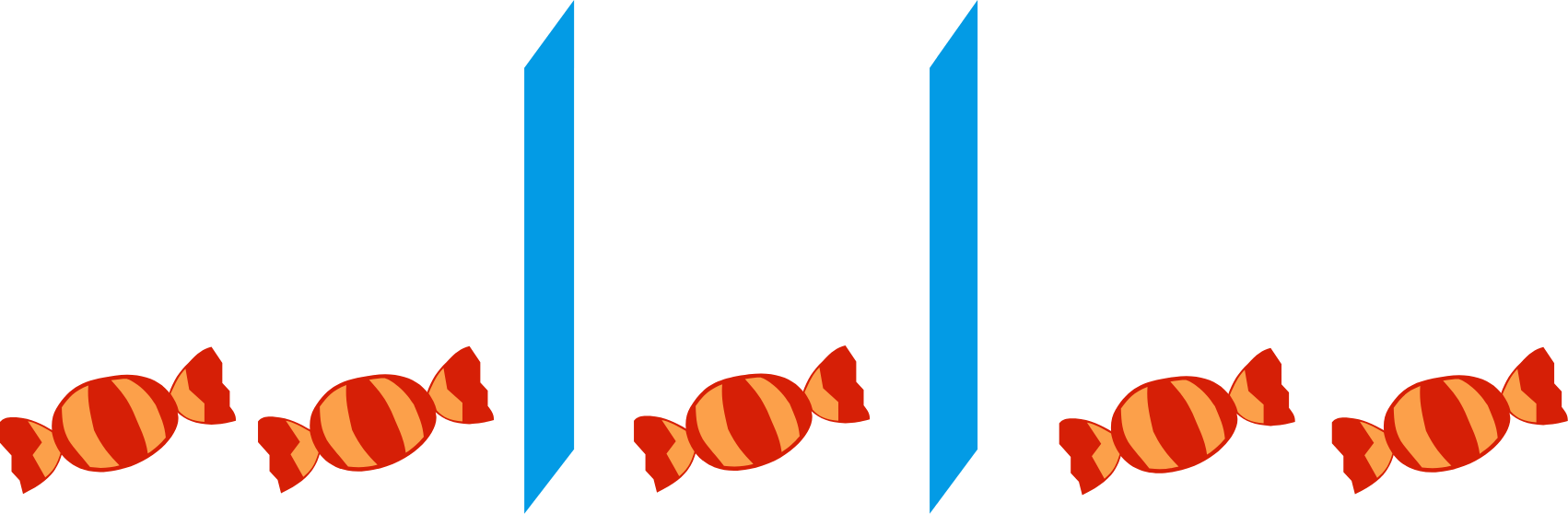Contest Math II

An important problem-solving skill - especially for math contests - is figuring out how to frame (or reframe) a situation to make it easier to solve. This course will guide you through the thought processes needed to excel at this problem-solving art.Reframing Problems

Consider the problem of finding the sum of all positive factors of 72. Typically, factors are found by creating pairs (or using a factor tree). An inefficient method for summing all the factors would involve finding all these pairs, and then summing them together:

$\begin{array}{c} 1 \times 72 \\ 2 \times 36 \\ 3 \times 24 \\ \vdots \\ \text{Sum of factors}= 1+72+2+36+3+24+\cdots \end{array}$

However, there is a more efficient way of finding the factors by using the prime factorization $72=2^3 \cdot 3^2.$ There are only two prime factors, so we can list all of the factors with a multiplication grid:The sum of all the factors of 72 is just the sum of all the numbers in this grid, and there happens to be a quick way to add all these numbers together. Using this, what is the sum of all of the positive factors of $72?$

Reframing Problems

To find the sum of the factors of a number with more than two prime factors, we can't make a grid of factors, but we can extend the same idea to more dimensions.

What is the sum of the positive factors of $360 = 2^3 \cdot 3^2 \cdot 5?$

Reframing Problems

The art of reframing a problem is present across all topics. In the next few problems, we'll look at an application in Combinatorics: the branch of Discrete Math concerned with counting.Reframing Problems

How many ways are there to divide 5 indistinguishable candies among 3 distinguishable friends?Note that all candies must be distributed, and people can receive 0 candies.

Reframing Problems

In combinatorics problems, a re-framing can reveal an equivalent set of possibilities that is easier to count.

Consider the previous problem of distributing 5 indistinguishable candies among 3 distinguishable people. This was relatively tedious to count, especially because the people were distinguishable. For example, there are 3 ways to distribute all 5 candies to 1 person, but there are 6 ways to distribute 4 candies to 1 person and 1 candy to a different person.

However, we can re-frame this problem: We put the 5 candies in a line, and separate them into groups with two indistinguishable dividers.Each different way of placing the dividers gives a different way to distribute the candy. The above example corresponds to the distribution of 2 candies to the first person, 1 candy to the second person, and 2 candies to the third person. Thus, we now have a different, equivalent problem: How many ways are there to place the candies and dividers into a line? It turns out, there is a very simple way to compute this without resorting to casework or tedious counting.

Reframing Problems

In a fish tank, there are 4 distinct fish. You spread 10 identical food pellets into the tank.

How many different distributions of food pellets among the fish are possible, given that fish can receive 0 pellets and every pellet goes to some fish?Reframing Problems

We can generalize the answer from the previous problems. When distributing $n$ identical objects to $r$ distinguishable bins, it's equivalent to arranging $n$ identical objects and $r-1$ identical dividers, so it can be done in $\binom{n+r-1}{r-1}$ ways. However, in problem-solving, it's important to understand the method rather than to memorize the formula.

By doing so, you'll be able to tackle problems that you encounter that you haven't seen before by relating them to what you already know, and making the necessary modifications.

In the next problem, we'll see a slight modification on the previous fish feeding problem.

Reframing Problems

In a fish tank, there are 4 distinct fish. You spread 10 identical food pellets into the tank. However, you don't want any fish to go hungry, so each fish must receive at least 1 pellet.

How many different distributions of food pellets among the fish are possible?Reframing Problems

×